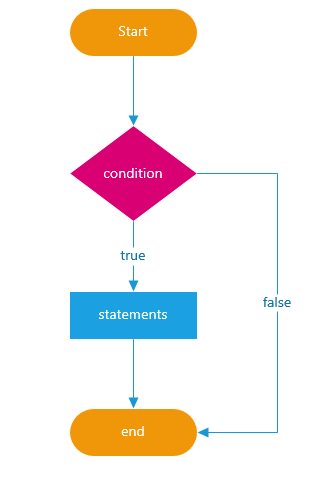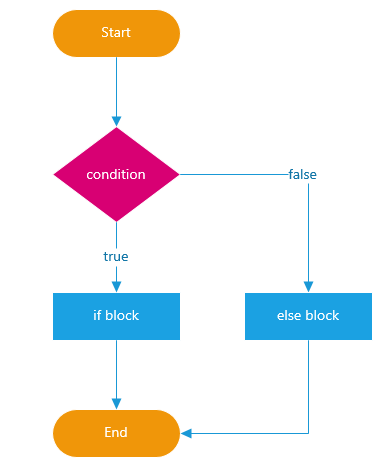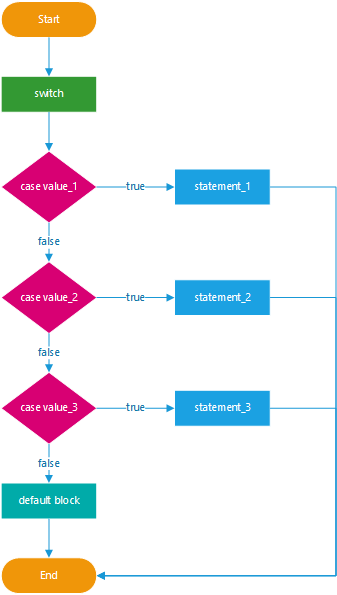# JavaScript Conditional Statements

## JavaScript Conditional Statements with Examples

In this article, I am going to discuss JavaScript Conditional Statements with Examples. Please read our previous article where we discussed JavaScript Operators in Detail. At the end of this article, you will understand what are JavaScript Conditional Statements and when and how to use this in JavaScript with examples.

##### What are JavaScript Conditional Statements?

Condition is test for something. It’s a very important for programming in a several ways. The Condition is all about making decision. Conditional statements are used to execute the code based on different conditions. If a condition is true, we can perform one action and if the condition is false, we can perform another action.

Conditional statements in JavaScript are:

• if statement
• if…else statement
• if…else if statement
• switch statement
##### if statement in JavaScript

Use if statement, if we want to execute a set of code in case the given condition is true. The following flowchart describes the if statement.##### Syntax to use if statement in javaScript:

Following is the syntax to use the if conditional statement in JavaScript.

```if(condition)
{
code to be executed if condition is true
}```
##### If Statement Example in JavaScript:

Following is an example to understand the if conditional statement in JavaScript.

```<html>
<body>
<script type=”text/javascript”>
var a,b;
a=60
b=30
document.write("<br>Value of a "+a);
document.write("<br>Value of b "+b);
if (a>b)
{
document.write("<br>value of a is greater than b ");
}
</script>
</body>
</html>```
##### if…else statement in JavaScript

Use if…else statement, if we want to execute a set of code in case the given condition is true and other code in case of given condition is false. The following flowchart describes the if else statement.##### Syntax:
```if(condition)
{
code to be executed if condition is true
}
else
{
code to be executed if condition is false
}```
##### Example:
```<script type="text/javascript">
var a, b;
a = 60
b = 30
document.write("<br>Value of a " + a);
document.write("<br>Value of b " + b);
if (a > b) {
document.write("<br>value of a is greater than b");
}
else {
document.write("<br>value of a is less than b");
}
</script>```

Note: Always use {… } even if there is only one statement to be executed, this makes code look good, easier to read and avoid any ambiguity

##### if…else if statement

Use this if we want to execute a bunch of codes.

##### Syntax:
```if(condition1)
{
code to be executed if condition1 is true
}
else if(condition2)
{
code to be executed if condition1 is false and condition2 one is true
}
else
{
code to be executed if both condition1 and condition2 is false
}```
##### Example:
```<script type="text/javascript">
var a, b;
a = 60
b = 30
document.write("<br>Value of a " + a);
document.write("<br>Value of b " + b);
if (a > b) {
document.write("<br>value of a is greater than b");
}
else if (a < b) {
document.write("<br>value of a is less than b");
}
else {
document.write("<br>value of a is equal to b");
}
</script>```
##### Switch statement in JavaScript

Use this, if we want to execute bunch of codes for different condition. The switch statement is similar to if…else statement. The following flowchart describes the switch statement.##### Syntax:
```switch(expression)
{
case 1 :
code to be executed
break
case 2 :
code to be executed
break
case 3 :
code to be executed
break
default :
code to be executed if none of the above given cases are true
}```
##### How switch-case works?
1. The expression is evaluated
2. When one of the constants specified in a case label is equal to the expression
• The statement that corresponds to that case is executed
3. If no case is equal to the expression
• If there is default case, it is executed
• Otherwise the control is transferred to the end point of the switch statement
4. The break statement exits the switch-case statement
##### Example:
```<script type="text/javascript">
var day;
weekday = parseInt(prompt("Enter Weekday", ""))
switch (weekday) {
case 1:
day = "Sunday";
break
case 2:
day = "Monday";
break
case 3:
day = "Tuesday";
break
case 4:
day = "Wednesday";
break
case 5:
day = "Thursday";
break
case 6:
day = "Friday";
break
case 7:
day = "Saturday";
break
default:
day = "Invalid weekday";
}
</script>```